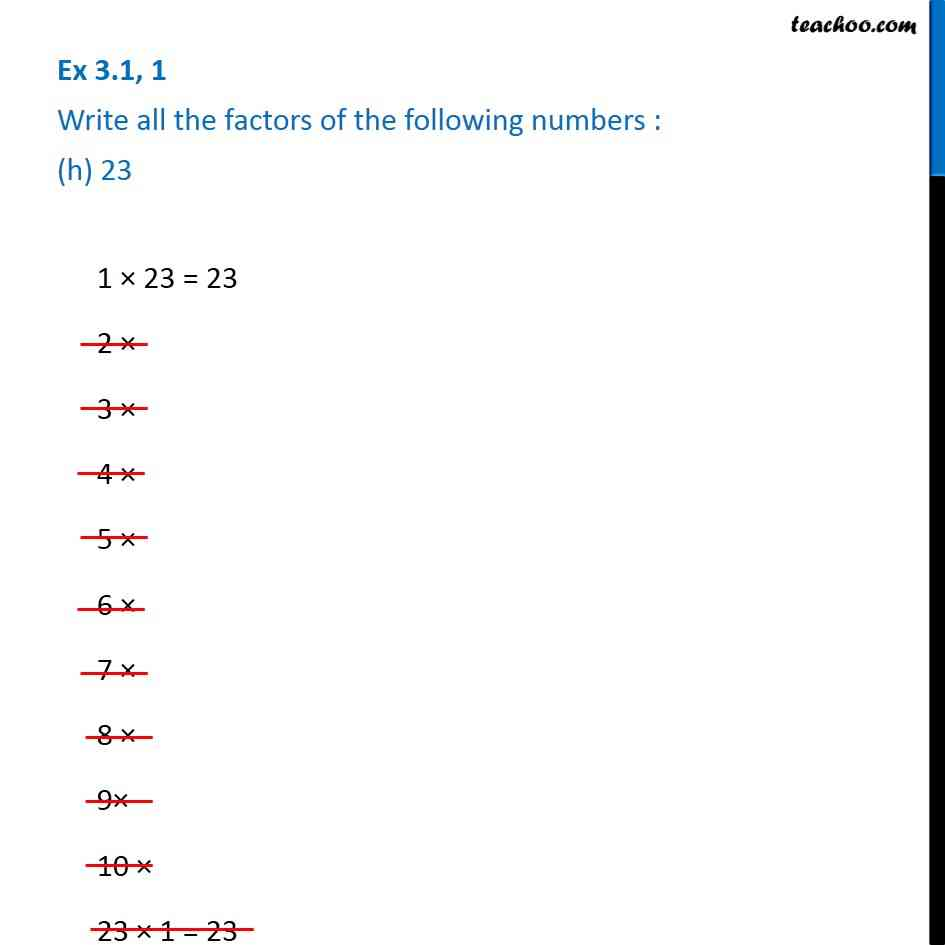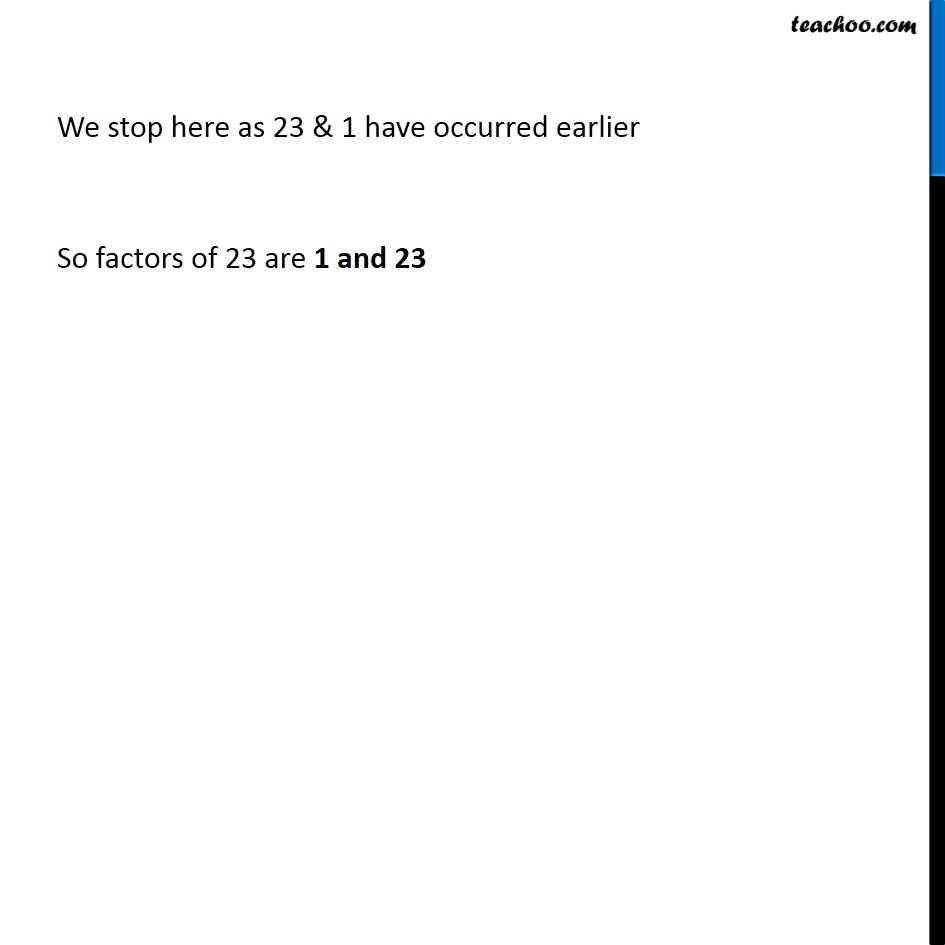1. Chapter 3 Class 6 Playing with Numbers
2. Serial order wise
3. Ex 3.1

Transcript

Ex 3.1, 1 Write all the factors of the following numbers : (h) 23 1 × 23 = 23 2 × 3 × 4 × 5 × 6 × 7 × 8 × 9× 10 × 23 × 1 = 23 We stop here as 23 & 1 have occurred earlier So factors of 23 are 1 and 23